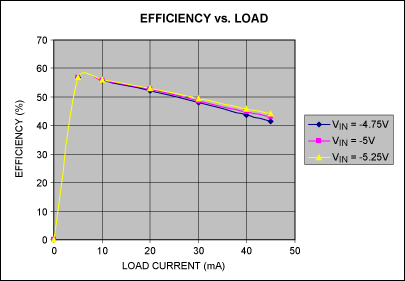# Negative Step-Down Converter Requires No Inductor

### Abstract

This circuit uses two charge-pump devices to step down a negative voltage. The first produces a positive output by doubling and inverting the negative input voltage, and the second acts as an inverter to produce the desired negative output. Input and output capabilities for the circuit depend on the input/output voltages allowed by the IC components chosen.

A similar version of this article appeared in the May 7, 2009 issue of Electronic Design magazine.

To step down a negative voltage in a low-current application, a non-inductor configuration combines ease of use with a low number of external components. Such step-down converters can be implemented with two charge-pump devices. The first produces a positive output by doubling and inverting the negative input voltage, and the second acts as an inverter to produce the desired negative output. Input and output capabilities for the circuit depend on the input/output voltages allowed by the IC components chosen (Figure 1).U1 (MAX1682) operates as a voltage doubler that accepts the negative 5V input and produces a positive 5V output. A regulated voltage inverter (U2, MAX1673) then accepts the +5V and produces a -3V output. A voltage divider at the U2 output (R1/R2) provides feedback for regulating U2's output voltage to the desired level (VOUT). At the FB input, the threshold voltage is factory-set to zero. You can choose the values for R1 and R2 using R2(5V/R1) = -VOUT, plus the condition that their sum should allow a minimum current flow of 50µA. The accuracy of VOUT depends largely on the accuracy of the -5V input. Figures 2 and 3 depict the circuit efficiency and output regulation for inputs of -4.75V, -5V, and -5.25V.